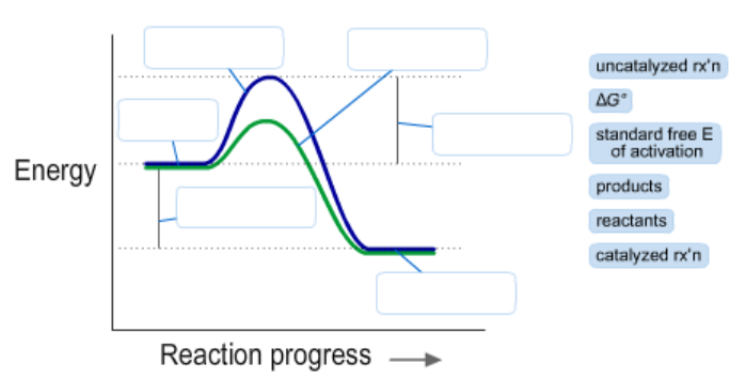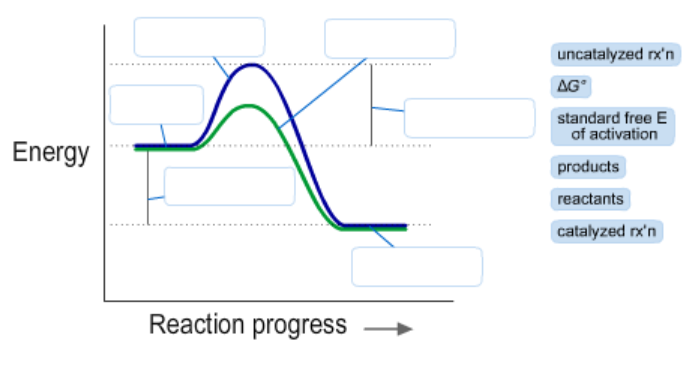# Problem: The diagram below represents a spontaneous reaction (deltaG degree &lt; 0). Drag the labels to the correct bins.

###### FREE Expert Solution

We’re being asked to label the given energy diagram.Recall that an energy diagram is usually read from left to right

The components of energy diagram are:

93% (50 ratings)###### Problem Details

The diagram below represents a spontaneous reaction (deltaG degree < 0). Drag the labels to the correct bins.